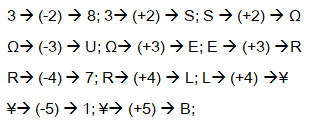# Reasoning Questions (Alpha Numeric & Number Sequence)

Practice Reasoning Questions For IBPS RRB 2017 (Alpha Numeric Symbols &amp; Number Sequence):

Dear Readers, Important Practice Reasoning Questions with explanation for IBPS Exams 2017 was given here with explanation, candidates those who are preparing for Banking and all other Competitive exams can use this practice questions.

Direction (Q.1-5): Study the following information carefully and answer the questions given below.

\$ 5 G A H 8 # 3 U S 9 Ω W 7 E J Ψ R % Q 1 L 2 I N ¥ 4 © 6 O B

1). If all the symbols are dropped from the above arrangement then which of the following will be thirteenth element from the right end of the given arrangement?

1. E
2. 7
3. J
4. R
5. 9

2). What should be in the place of question mark (?) in the following series based on the given arrangement?

38S           ΩUE              R7L     ?

1. ¥LO
2. NQO
3. ¥1B
4. NL6
5. None of these

3). Which of the following is fourth to the right of the nineteenth element from the left end of the given arrangement?

1. W
2. L
3. %
4. 2
5. U

4). How many such vowels are there in the arrangement each of which immediately followed by a consonant and also immediately notpreceded by a number?

1. One
2. Two
3. Three
4. More than three
5. None

5). How many such numbers are there in the arrangement each of which immediately preceded by letter and immediately followed by symbol?

1. None
2. One
3. Two
4. Three
5. More than three

Direction (Q. 6-8): Following questions are based on the five numbers given below.Study the questions carefully and answer it.

457     743     614     298     329

6). If the positions of the first and the third digits in each of the above numbers are interchanged, which of the following will be the third highest number?

1. 298
2. 614
3. 743
4. 457
5. None of these

7). If in each number, the digits are arranged in ascending order within the number, what will be the sum of the middle digits of the highest number and the second lowest number?

1. 13
2. 7
3. 8
4. 9
5. None of these

8). If the position of the second and the third digits in each of the above numbers are interchanged, which of the following will be the second highest number?

1. 457
2. 743
3. 614
4. 298
5. 329

Direction (Q. 9-10): Following questions are based on the five numbers given below. Study the questions carefully and answer it.

729     867     308     652     460

9). If in each number, ‘one’ is added to every even digits and ‘two’ is subtracted from every odd digits and put ‘one’ for ‘zero’, then what is the difference between the middle digit of second number from right end and the last digit of second number from the left end?

1. 4
2. 2
3. 3
4. 1
5. None of these

10). If in each number, the digits are arranged in descending order, what will be the sum of the middle digits of each of the numbers?

1. 26
2. 25
3. 33
4. 31
5. None of these

Explanation:

Question (1-5)

\$ 5 G A H 8 # 3 U S 9 Ω W 7 E J Ψ R % Q 1 L 2 I N ¥ 4 © 6 O B

Let drop the all the symbols in the arrangement,

5 G A H 8 3 U S 9 W 7 E J R Q 1 L 2 I N 4 6 O B

Then, 13th element from the right end is ‘E’

38S     ΩUE              R7L     ?Hence, the answer is ‘¥ 1 B’

Fourth to the right of the nineteenth element from the left end i.e. 19 + 4 = 23rd element from the left end = ‘2’

\$ 5 G A H 8 # 3 U S 9 Ω W 7 E J Ψ R % Q 1 L 2 I N ¥ 4 © 6 O B

There is only one such a vowel.

\$ 5 G A H 8 # 3 U S 9 Ω W 7 E J Ψ R % Q 1 L 2 I N ¥ 4 © 6 O B

There are two such a numbers.

Question (6-8):

457     743     614     298     329

Interchanging the first and third digits of the each numbers we get,

754     347     416     892     923

∴ the third highest number is ‘754’.

Hence, the corresponding number is 457.

Ascending order of the digits,

457     347     146     289     239

The highest number is, 457

The second lowest number is 289

Hence, required sum = 5 + 8 = 13

Interchanging the second and third digits of each numbers, we get

475     734     641     289     392

∴ the second highest number is, 641

Hence, the corresponding number is 614

Question (9-10):

729     867     308     652     460

Adding to ‘one’ to the every even digits and subtracting ‘two’ from every odd digits, we get 537            975     119     733     571

The second number from the right end is 733

The second number from left end is 975

Hence, the required difference = 5 – 3 = 2